Rheonics SRV viscosity measurements can be converted to cup seconds, for different cup types, and for small viscosity variations.

We assume that there is a linear relationship between the cup second measurements and the dynamic viscosity in cP (mPa.s).

Therefore, we can simplify the relationship as: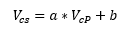(1)

Where a and b are the slope and offset between the two respectively.

For different cup types we have found the following relationships between cup seconds measurements and dynamic viscosity in cP:

 Cup Type a b ISO 4mm 0.76 2 Afnor 4 0.14 36 BS B4 0.29 9 Ford 4 0.24 8 Zahn 4 0.09 -2 Din 4 0.23 5

Table 1 Cup second conversion coefficients

## Calculating Custom Coefficients:

If your cup is not listed in the table above, then you can use the specifications sheet of the cup to find a relationship between cup seconds measurements and cP. You could also find the direct relationship empirically between the SRV and the cup.

In this case, “a” is defined as: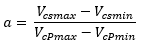(2)

And “b” can be found by selecting the maximum or minimum over the measurement range.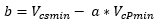(3)

### Finding coefficients from specifications:

You can find different conversion tables online or from the cup manufacturer. For example, in the table below, the equivalencies between the cup seconds and centipoise can be seen for a measurement range: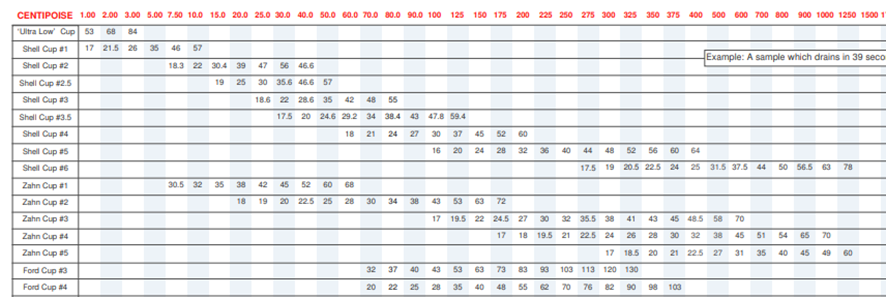Figure 1 Cup second equivalencies – these are generally valid for a certain type of viscometer so they are not universal.

For example. If we use Shell cup #3 over the measurement range of 18.6 to 28.6 seconds, the viscosities in cP over that range are 25 to 40 cP respectively.

Replacing these values in equation (2) we can find coefficient a: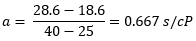(4)

And replacing result of equation (4) in equation (3), we can find coefficient b.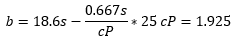(5)

### Finding Coefficients Experimentally

Instead of using the vendor specifications for cup conversion you can directly use the SRV measurements and cup second measurements over a range.

1. Make sure that the temperature doesn’t vary during the process.
2. For lower range measure Dynamic Viscosity with SRV and the cup seconds viscosity. Take note of both values. This will be VcPmin and Vcsmin respectively.
3. For highest range measure Dynamic Viscosity with SRV and the cup seconds viscosity. Take note of both values. This will be VcPmax and Vcsmax respectively.
4. Replace these values in equations (2) and (3), as we did in previous section, to find the “a” and “b” coefficients.

 IMPORTANT: We are assuming that over the measurements range, the temperature remains constant and that the relationship between cup seconds and cP is linear. HOWEVER, in most processes, the temperature doesn’t remain constant, and viscosity can be highly dependent on it. This WILL affect the calculated coefficients a, and b. Also, the relationship between cup seconds and cP is usually non-linear during the entire measurements range of the cup. Following the non-linear dependency: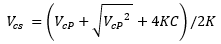Therefore, we encourage you to use the measurements from SRV, instead of making the cup-seconds conversions.

## Analyzing the viscosity variations:

For a certain measurement range, and from equation (1), we can deduce that a viscosity variation in cup seconds correlates with a viscosity variation in cP as follows: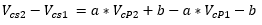(6)

And simplifying equation (6) we get: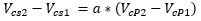(7)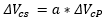(8)

Having a linear relationship between the two, with a constant slope a.

From equation 8 we can deduce that a percentile variation within a measurement range in cP relates with the same percentile cup second variation as follows.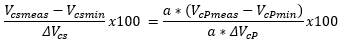(9)

Simplifying equation (9) we get: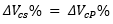(10)

This is; a percentile variation of measured viscosity within a range in cP is equivalent to the variation in cup seconds, for small variation ranges.

## Example: Ford 4 cup.

Measuring 94 cP with SRV (assuming that density = 1 g/cc) and applying equation (1) and the table coefficients, we obtain: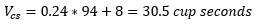(11)